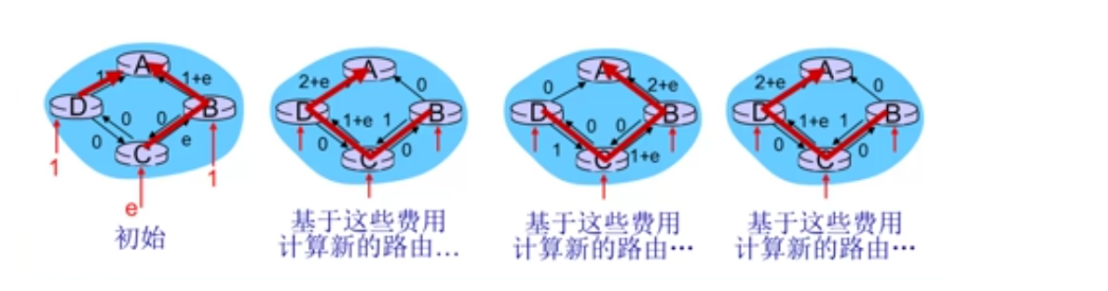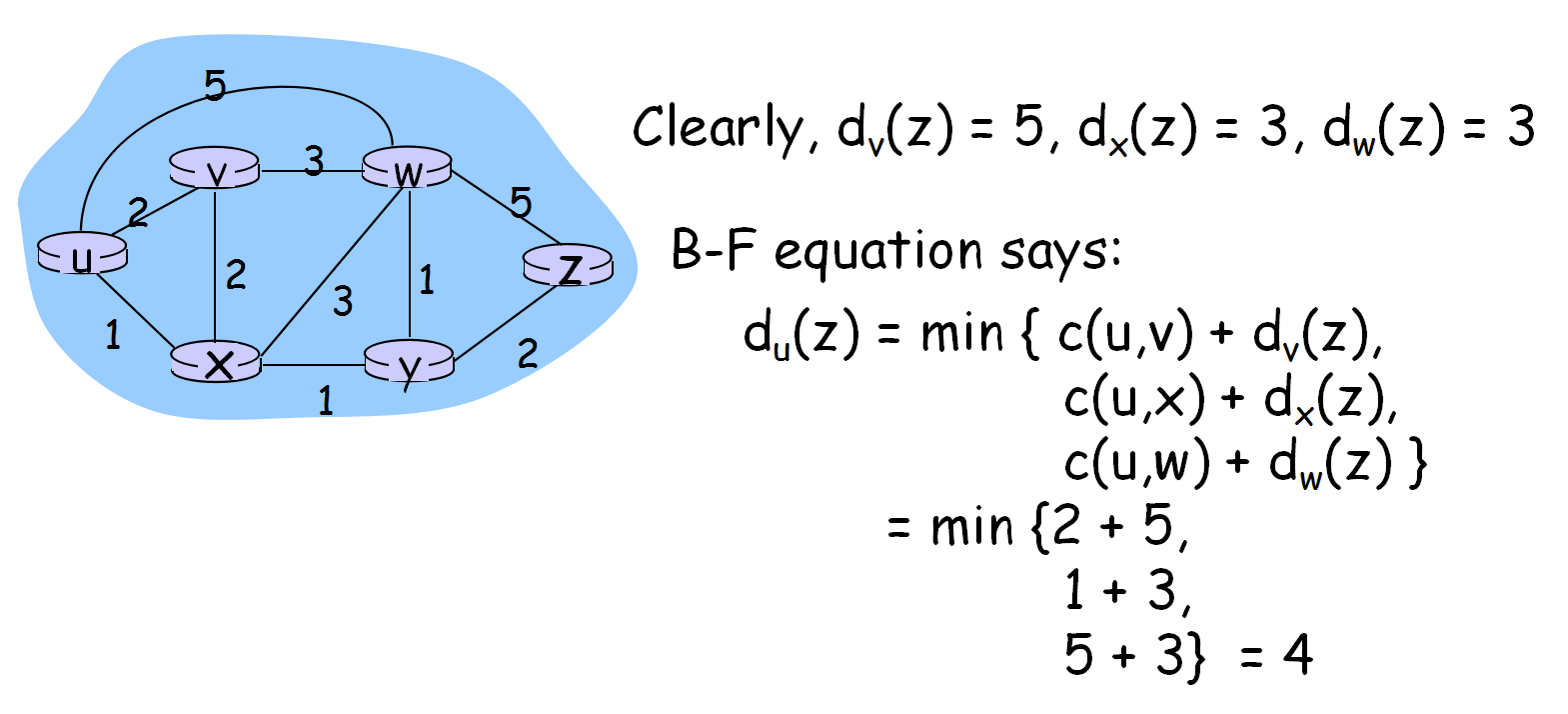# 计算机网络最佳路由表算法,计算机网络 路由算法1、路由算法概述

N=路由器集合，E=链路集合

2、路由算法分类

3、链路状态路由算法

Dijkstra算法

通过链路状态广播。

所有结点拥有相同信息。

获得该结点的转发表

c(x,y) 结点x到y的链路费用，不直接相连为记为无穷大

D(v) 从源到目的v的当前路径费用值

p(v) 沿从源到v的当前路径，v的前序结点

N' 已经找到最小费用路径的结点集合

★算法描述：

1  Initialization: 2    N' = {u} 3    for all nodes v 4      if v adjacent to u 5          then D(v) = c(u,v) 6      else D(v) = ∞ 7 8   Loop 9     find w not in N' such that D(w) is a minimum 10    add w to N' 11    update D(v) for all v adjacent to w and not in N' : 12       D(v) = min( D(v), D(w) + c(w,v) ) 13    /* new cost to v is either old cost to v or known 14     shortest path cost to w plus cost from w to v */

15  until all nodes in N'

★算法复杂性：n个结点

每次迭代需要检测所有不再集合N'中的结点w

n(n+1)/2次比较：O(n2)

更高效的实现：O（nlogn）

★存在问题：震荡（oscillations）4、距离向量算法

Bellman-Ford方程（动态规划）

Definedx(y) := cost of least-cost path from x to yThendx(y) = min {c(x,v) + dv(y) }where min is taken over all neighbors v of xDx(y)=从结点x到结点y的最小费用估计

x维护距离向量（DV）：Dx=[Dx(y): y∈N]

已知到达每个邻居的费用：c(x,v)

维护其所有邻居的距离向量：Dv=[Dv(y): y∈N]

每个结点不定时地将其自身的DV估计发送给其邻居。

当x接收到邻居的新的DV估计时，即一句B-F更新其自身的距离向量估计

Dx(y) ← minv{c(x,v) + Dv(y)}    for each node y ∊ N

Dx(y)最终会收敛于实际的最小费用dx(y)

1、异步迭代：

引发每次局部迭代的因素

1）局部链路费用改变

2）来自邻居的DV变化

2、分布式

每个结点只有当DV变化时才通告给邻居，邻居在必要时（其DV更新后发生变化）再通告它们的邻居。

↓

↓

（回到等待……）

1、毒性逆转

2、定义最大度量

--------------------------------------------------------------